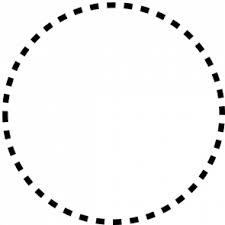# Dots In A Circle

Probability Level 3There are 40 dots spaced evenly in a circle. If 4 distinct dots $W, X, Y$ and $Z$ are picked randomly, what is the probability that $WX$ intersects $YZ$?

If the probability can be expressed as $\dfrac ab$, where $a$ and $b$ are coprime positive integers, find $a+b$.

×